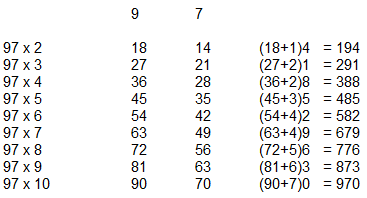## Learn Multiplication Tricks#### How to write Table of any two digit numbers ?

You can very easily multiply any two digit numbers (10-99) in a very easy manner using the following tricks.

Lets take table of 97

97 x 1 = 97

97 x 2 = 194

97 x 3 = 291

97 x 4 = 388

97 x 5 = 485

97 x 6 = 582

97 x 7 = 679

97 x 8 = 776

97 x 9 = 873

97 x 10 = 970

Here we implement the trick and find the solution in easy way

97 x 8

Take 9 and 7 separately and multiply 9 and 7 with 8

9 x 8 and 7 x 8

Then we get the following:

72 and 56

The add first result with first digit of second (72+5) and join with next digit(1) that is 776

(72+5) 6 = 776

In this way we can multiply whole table....try this trick on other tables too....

NEXT.....What is a Proxy Server